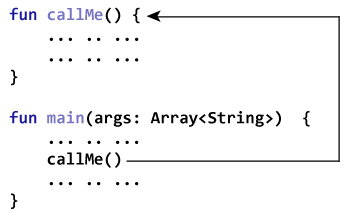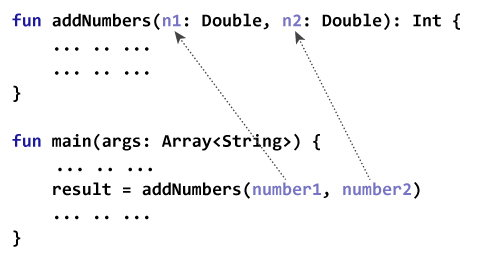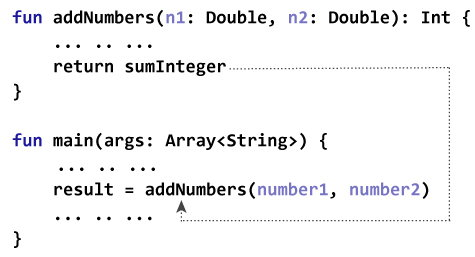# Kotlin Functions

In this article, you'll learn about functions; what functions are, its syntax and how to create a user-function in Kotlin.

In programming, function is a group of related statements that perform a specific task.

Functions are used to break a large program into smaller and modular chunks. For example, you need to create and color a circle based on input from the user. You can create two functions to solve this problem:

• `createCircle()` Function
• `colorCircle()` Function

Dividing a complex program into smaller components makes our program more organized and manageable.

Furthermore, it avoids repetition and makes code reusable.

## Types of Functions

Depending on whether a function is defined by the user, or available in standard library, there are two types of functions:

• Kotlin Standard Library Function
• User-defined functions

### Kotlin Standard Library Function

The standard library functions are built-in functions in Kotlin that are readily available for use. For example,

• `print()` is a library function that prints message to the standard output stream (monitor).
• `sqrt()` returns square root of a number (`Double` value)
``````fun main(args: Array<String>) {

var number = 5.5
print("Result = \${Math.sqrt(number)}")
}``````

When you run the program, the output will be:

`Result = 2.345207879911715`

Here is a link to the Kotlin Standard Library for you to explore.

### User-defined Functions

As mentioned, you can create functions yourself. Such functions are called user-defined functions.

### How to create a user-defined function in Kotlin?

Before you can use (call) a function, you need to define it.

Here's how you can define a function in Kotlin:

```fun callMe() {
// function body
}
```

To define a function in Kotlin, `fun` keyword is used. Then comes the name of the function (identifier). Here, the name of the function is callMe.

In the above program, the parenthesis `( )` is empty. It means, this function doesn't accept any argument. You will learn about arguments later in this article.

The codes inside curly braces `{ }` is the body of the function.

### How to call a function?

You have to call the function to run codes inside the body of the function. Here's how:

`callme()`

This statement calls the `callMe()` function declared earlier.### Example: Simple Function Program

``````fun callMe() {
println("Printing from callMe() function.")
println("This is cool (still printing from inside).")
}

fun main(args: Array<String>) {
callMe()
println("Printing outside from callMe() function.")
}``````

When you run the program, the output will be:

```Printing from callMe() function.
This is cool (still printing from inside).
Printing outside from callMe() function.```

The `callMe()` function in the above code doesn't accept any argument.

Also, the function doesn't return any value (return type is `Unit`).

Let's take another function example. This function will accept arguments and also returns a value.

### Example: Add Two Numbers Using Function

``````fun addNumbers(n1: Double, n2: Double): Int {
val sum = n1 + n2
val sumInteger = sum.toInt()
return sumInteger
}

fun main(args: Array<String>) {
val number1 = 12.2
val number2 = 3.4
val result: Int

println("result = \$result")
}``````

When you run the program, the output will be:

`result = 15`

### How functions with arguments and return value work?

Here, two arguments number1 and number2 of type `Double` are passed to the `addNumbers()` function during function call. These arguments are called actual arguments.

`result = addNumbers(number1, number2)`

The parameters n1 and n2 accepts the passed arguments (in the function definition). These arguments are called formal arguments (or parameters).In Kotlin, arguments are separated using commas. Also, the type of the formal argument must be explicitly typed.

Note that, the data type of actual and formal arguments should match, i.e., the data type of first actual argument should match the type of first formal argument. Similarly, the type of second actual argument must match the type of second formal argument and so on.

Here,

`return sumInteger`

is the return statement. This code terminates the `addNumbers()` function, and control of the program jumps to the `main()` function.

In the program, sumInteger is returned from `addNumbers()` function. This value is assigned to the variable result.Notice,

• both sumInteger and result are of type `Int`.
• the return type of the function is specified in the function definition.
```// return type is Int
fun addNumbers(n1: Double, n2: Double): Int {
... .. ...
}```

If the function doesn't return any value, its return type is `Unit`. It is optional to specify the return type in the function definition if the return type is `Unit`.

### Example: Display Name by Using Function

``````fun main(args: Array<String>) {
println(getName("John", "Doe"))
}

fun getName(firstName: String, lastName: String): String = "\$firstName \$lastName"``````

When you run the program, the output will be:

`John Doe`

Here, the `getName()` function takes two `String` arguments, and returns a `String`.

You can omit the curly braces `{ }` of the function body and specify the body after `=` symbol if the function returns a single expression (like above example).

It is optional to explicitly declare the return type in such case because the return type can be inferred by the compiler. In the above example, you can replace

`fun getName(firstName: String, lastName: String): String = "\$firstName \$lastName"`

with

```fun getName(firstName: String, lastName: String) = "\$firstName \$lastName"
```

This is just the brief introduction to functions in Kotlin. Recommended articles related to functions:

• Kotlin inline functions
• Kotlin infix functions
• Kotlin function scope
• Kotlin Default and Named Arguments
• Kotlin Recursion
• Kotlin Tail Recursive function
• Kotlin Extension Function
• Kotlin High-Order Functions & Lambdas